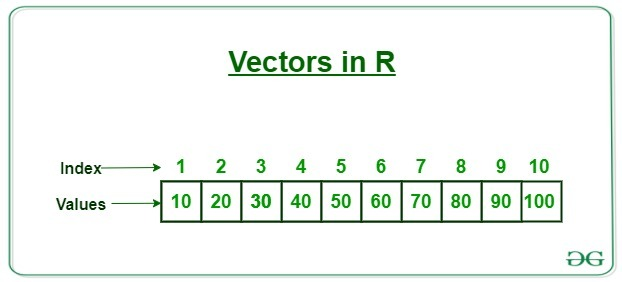Open in App
Not now

# R – Vector

• Difficulty Level : Easy
• Last Updated : 22 Apr, 2020

R programming is one of the most popular languages when it comes to data science, statistical computations or scientific research. R programming is widely used in machine learning and it is very efficient and user-friendly. It provides flexibility in doing big statistical operations with a few lines of code.

Vectors in R are the same as the arrays in C language which are used to hold multiple data values of the same type. One major key point is that in R the indexing of the vector will start from ‘1’ and not from ‘0’. We can create numeric vectors and character vectors as well.#### Types of vectors

Vectors are of different types which are used in R. Following are some of the types of vectors:

• Numeric vectors
Numeric vectors are those which contain numeric values such as integer, float, etc.

 `# R program to create numeric Vectors ` ` `  `# creation of vectors using c() function. ` `v1 <``-` `c(``4``, ``5``, ``6``, ``7``) ` ` `  `# display type of vector ` `typeof(v1) ` ` `  `# by using 'L' we can specify that we want integer values. ` `v2 <``-` `c(``1L``, ``4L``, ``2L``, ``5L``)  ` ` `  `# display type of vector ` `typeof(v2) `

Output:

``` "double"
 "integer"```
• Character vectors
Character vectors contain alphanumeric values and special characters.

 `# R program to create Character Vectors ` ` `  `# by default numeric values  ` `# are converted into characters ` `v1 <``-` `c(``'geeks'``, ``'2'``, ``'hello'``, ``57``)  ` ` `  `# Displaying type of vector ` `typeof(v1) `

Output:

` "character"`
• Logical vectors
Logical vectors contain boolean values such as TRUE, FALSE and NA for Null values.

 `# R program to create Logical Vectors ` ` `  `# Creating logical vector ` `# using c() function ` `v1 <``-` `c(TRUE, FALSE, TRUE, NA) ` ` `  `# Displaying type of vector ` `typeof(v1) `

Output:

` "logical"`

#### Creating a vector

There are different ways of creating vectors. Generally, we use ‘c’ to combine different elements together.

 `# R program to create Vectors ` ` `  `# we can use the c function ` `# to combine the values as a vector. ` `# By default the type will be double ` `X <``-` `c(``61``, ``4``, ``21``, ``67``, ``89``, ``2``) ` `cat(``'using c function'``, X, ``'\n'``) ` ` `  `# seq() function for creating ` `# a sequence of continuous values. ` `# length.out defines the length of vector. ` `Y <``-` `seq(``1``, ``10``, length.out ``=` `5``)  ` `cat(``'using seq() function'``, Y, ``'\n'``)  ` ` `  `# use':' to create a vector  ` `# of continuous values. ` `Z <``-` `2``:``7` `cat(``'using colon'``, Z) `

Output:

```using c function 61 4 21 67 89 2
using seq() function 1 3.25 5.5 7.75 10
using colon 2 3 4 5 6 7
```

#### Accessing vector elements

Accessing elements in a vector is the process of performing operation on an individual element of a vector. There are many ways through which we can access the elements of the vector. The most common is using the ‘[]’, symbol.

Note: Vectors in R are 1 based indexing unlike the normal C, python, etc format.

 `# R program to access elements of a Vector ` ` `  `# accessing elements with an index number. ` `X <``-` `c(``2``, ``5``, ``18``, ``1``, ``12``) ` `cat(``'Using Subscript operator'``, X[``2``], ``'\n'``) ` ` `  `# by passing a range of values ` `# inside the vector index. ` `Y <``-` `c(``4``, ``8``, ``2``, ``1``, ``17``) ` `cat(``'Using combine() function'``, Y[c(``4``, ``1``)], ``'\n'``) ` ` `  `# using logical expressions ` `Z <``-` `c(``5``, ``2``, ``1``, ``4``, ``4``, ``3``) ` `cat(``'Using Logical indexing'``, Z[Z>``4``]) `

Output

```Using Subscript operator 5
Using combine() function 1 4
Using Logical indexing 5
```

#### Modifying a vector

Modification of a Vector is the process of applying some operation on an individual element of a vector to change its value in the vector. There are different ways through which we can modify a vector:

 `# R program to modify elements of a Vector ` ` `  `# Creating a vector ` `X <``-` `c(``2``, ``7``, ``9``, ``7``, ``8``, ``2``) ` ` `  `# modify a specific element ` `X[``3``] <``-` `1` `X[``2``] <``-``9` `cat(``'subscript operator'``, X, ``'\n'``) ` ` `  `# Modify using different logics. ` `X[X>``5``] <``-` `0` `cat(``'Logical indexing'``, X, ``'\n'``) ` ` `  `# Modify by specifying  ` `# the position or elements. ` `X <``-` `X[c(``3``, ``2``, ``1``)] ` `cat(``'combine() function'``, X) `

Output

```subscript operator 2 9 1 7 8 2
Logical indexing 2 0 1 0 0 2
combine() function 1 0 2
```

#### Deleting a vector

Deletion of a Vector is the process of deleting all of the elements of the vector. This can be done by assigning it to a NULL value.

 `# R program to delete a Vector ` ` `  `# Creating a Vector ` `M <``-` `c(``8``, ``10``, ``2``, ``5``) ` ` `  `# set NULL to the vector ` `M <``-` `NULL  ` `cat(``'Output vector'``, M) `

Output:

```Output vector NULL
```

#### Sorting elements of a Vector

`sort()` function is used with the help of which we can sort the values in ascending or descending order.

 `# R program to sort elements of a Vector ` ` `  `# Creation of Vector ` `X <``-` `c(``8``, ``2``, ``7``, ``1``, ``11``, ``2``) ` ` `  `# Sort in ascending order ` `A <``-` `sort(X) ` `cat(``'ascending order'``, A, ``'\n'``) ` ` `  `# sort in descending order  ` `# by setting decreasing as TRUE ` `B <``-` `sort(X, decreasing ``=` `TRUE) ` `cat(``'descending order'``, B) `

Output:

```ascending order 1  2  2  7  8 11
descending order 11  8  7  2  2  1
```

My Personal Notes arrow_drop_up
Related Articles